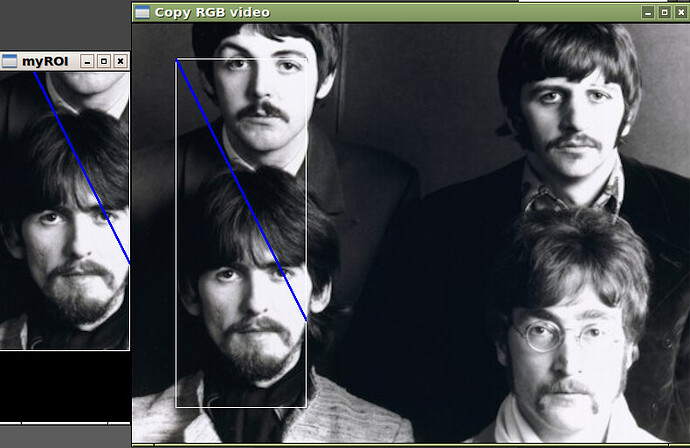# ROI image is not centered

Hello everyone.

I do not know if this is the right place to post my question, anyway, here it is. I’m trying to display a ROI from an OpenCV Mat structure. The ROI is displayed as it is shown in the attached image. The ROI is the white rectangle in the original image in the right. The problem is that a considerable part of the top of the ROI is missing and there is black space below. However, when the window is maximized, the complete ROI image is there. The code I use is posted below.

best wishes.

################

``````// HHAA
bool glfwNamedWindow(const std::string winName){

static GLenum err;

if (!display_windows){
return true;
}

GLFWwindow* window = glfwCreateWindow(window_width,
window_height, winName.c_str(), NULL, NULL);
if (window == NULL) {
glfwTerminate();
cout << "Error creating the window " << winName << glewGetErrorString(err) << endl;
std::cout.flush();
exit(EXIT_FAILURE);
}

windowList.push_back(window);
windowName.push_back(winName);

glfwSetKeyCallback(windowList[windowList.size() - 1], key_callback);
//glfwSetWindowSizeCallback(windowList[windowList.size() - 1], resize_callback);

glfwMakeContextCurrent(windowList[windowList.size() - 1]);
glfwSwapInterval(1);

// First time
if (windowList.size() == 1){

//  Initialise glew (must occur AFTER window creation or glew will error)
err = glewInit();
if (GLEW_OK != err){
cout << "GLEW initialisation error: " << glewGetErrorString(err) << endl;
std::cout.flush();
exit(-1);
}
cout << "GLEW okay - using version: " << glewGetString(GLEW_VERSION) << endl;

}

glViewport(0, 0, window_width, window_height); // use a screen size of WIDTH x HEIGHT

glMatrixMode(GL_PROJECTION);     // Make a simple 2D projection on the entire window
glOrtho(0.0, window_width, window_height, 0.0, 0.0, 100.0);

glMatrixMode(GL_MODELVIEW);    // Set the matrix mode to object modeling

glClearColor(0.0f, 0.0f, 0.0f, 0.0f);
glClearDepth(0.0f);
glClear(GL_COLOR_BUFFER_BIT | GL_DEPTH_BUFFER_BIT); // Clear the window

return true;

}

// HHAA
bool glfwImageShow(const std::string winName, cv::Mat &frame) {

cv::Size frame_size = frame.size();

if (!display_windows)
return true;

GLFWwindow* window = getWindow(winName);
if (window == NULL){
cout << "Error: No window with the name " << winName << endl;
std::cout.flush();
return false;
}

glfwSetWindowSize(window, frame_size.width, frame_size.height);
//glfwSetWindowSize(window, 640, 480);
//if (frame.isSubmatrix() == true)
//  glfwSetWindowSize(window, 640, 480);
//else
glfwMakeContextCurrent(window);
glfwSwapInterval(1);

// Clear color and depth buffers
glClear(GL_COLOR_BUFFER_BIT | GL_DEPTH_BUFFER_BIT);
glMatrixMode(GL_MODELVIEW);     // Operate on model-view matrix

glEnable(GL_TEXTURE_2D);
GLuint image_tex = matToTexture(frame, GL_LINEAR_MIPMAP_LINEAR, GL_LINEAR, GL_CLAMP);

glTexCoord2i(0, 0); glVertex2i(0, 0);
glTexCoord2i(0, 1); glVertex2i(0, frame_size.height);
glTexCoord2i(1, 1); glVertex2i(frame_size.width, frame_size.height);
glTexCoord2i(1, 0); glVertex2i(frame_size.width, 0);
glEnd();

glDeleteTextures(1, &image_tex);
glDisable(GL_TEXTURE_2D);

glfwSwapBuffers(window);
glfwPollEvents();

return true;

}

// Function turn a cv::Mat into a texture, and return the texture ID as a GLuint for use
GLuint matToTexture(cv::Mat &mat, GLenum minFilter, GLenum magFilter, GLenum wrapFilter) {
// Generate a number for our textureID's unique handle
GLuint textureID;
glGenTextures(1, &textureID);

// Bind to our texture handle
glBindTexture(GL_TEXTURE_2D, textureID);

// Catch silly-mistake texture interpolation method for magnification
if (magFilter == GL_LINEAR_MIPMAP_LINEAR  ||
magFilter == GL_LINEAR_MIPMAP_NEAREST ||
magFilter == GL_NEAREST_MIPMAP_LINEAR ||
magFilter == GL_NEAREST_MIPMAP_NEAREST)
{
cout << "You can't use MIPMAPs for magnification - setting filter to GL_LINEAR" << endl;
std::cout.flush();
magFilter = GL_LINEAR;
}

// Set texture interpolation methods for minification and magnification
glTexParameteri(GL_TEXTURE_2D, GL_TEXTURE_MIN_FILTER, minFilter);
glTexParameteri(GL_TEXTURE_2D, GL_TEXTURE_MAG_FILTER, magFilter);

// Set texture clamping method
glTexParameteri(GL_TEXTURE_2D, GL_TEXTURE_WRAP_S, wrapFilter);
glTexParameteri(GL_TEXTURE_2D, GL_TEXTURE_WRAP_T, wrapFilter);

// Set incoming texture format to:
// GL_BGR       for CV_CAP_OPENNI_BGR_IMAGE,
// GL_LUMINANCE for CV_CAP_OPENNI_DISPARITY_MAP,
// Work out other mappings as required ( there's a list in comments in main() )
GLenum inputColourFormat = GL_BGR;
if (mat.channels() == 1)
{
inputColourFormat = GL_LUMINANCE;
}
``````

Hello everyone.

I’m trying to display a ROI from an OpenCV Mat structure. The ROI is displayed as it is shown in the attached image. The ROI is the white rectangle in biggest image. The problem is that the top of the ROI is missing and there is black space below. However, when the ROI window is maximized, the complete image is there. The code used is posted below.

best wishes.

``````    // https://stackoverflow.com/questions/16809833/opencv-image-loading-for-opengl-texture

// use fast 4-byte alignment (default anyway) if possible
glPixelStorei(GL_UNPACK_ALIGNMENT, (mat.step & 3) ? 1 : 4);

// set length of one complete row in data (doesn't need to equal image.cols)
glPixelStorei(GL_UNPACK_ROW_LENGTH, mat.step/mat.elemSize());
// https://www.khronos.org/registry/OpenGL-Refpages/es3.0/html/glPixelStorei.xhtml

glPixelStorei(GL_UNPACK_SKIP_PIXELS, 0);

glPixelStorei(GL_UNPACK_IMAGE_HEIGHT, 0);
glPixelStorei(GL_UNPACK_ALIGNMENT, 0);

// Create the texture
glTexImage2D(GL_TEXTURE_2D,     // Type of texture
0,                 // Pyramid level (for mip-mapping) - 0 is the top level
GL_RGB8,            // Internal colour format to convert to
mat.cols,          // Image width  i.e. 640 for Kinect in standard mode
mat.rows,          // Image height i.e. 480 for Kinect in standard mode
0,                 // Border width in pixels (can either be 1 or 0)
inputColourFormat, // Input image format (i.e. GL_RGB, GL_RGBA, GL_BGR etc.)
GL_UNSIGNED_BYTE,  // Image data type
mat.ptr());        // The actual image data itself

// If we're using mipmaps then generate them. Note: This requires OpenGL 3.0 or higher
if (minFilter == GL_LINEAR_MIPMAP_LINEAR  ||
minFilter == GL_LINEAR_MIPMAP_NEAREST ||
minFilter == GL_NEAREST_MIPMAP_LINEAR ||
minFilter == GL_NEAREST_MIPMAP_NEAREST)
{
glGenerateMipmap(GL_TEXTURE_2D);
}

return textureID;
}
``````Hello again, just in case it is useful for someone else, I just moved

``````glViewport(0, 0, frame_size.width, frame_size.height); // use a screen size of WIDTH x HEIGHT

glMatrixMode(GL_PROJECTION);     // Make a simple 2D projection on the entire window
right after calling `glfwMakeContextCurrent(window)` and now my images are well centered.